Refer to our Texas Go Math Grade 5 Answer Key Pdf to score good marks in the exams. Test yourself by practicing the problems from Texas Go Math Grade 5 Module 14 Assessment Answer Key.

Vocabulary

Choose the best term from the box.

Question 1.
The ___ is the point where the x-axis and y-axis meet. Its __ is 0, and its ____ is 0. (p. 511)
Answer: The origin is the point where the x-axis and y-axis meet. Its x is 0, and its y is 0.

Question 2.
The horizontal number line in a coordinate grid is called the ____. (p. 511)
Answer: The horizontal number line in a coordinate grid is called the x-axis.

Concepts and Skills

Use the table for 3-4. TEKS 5.8.C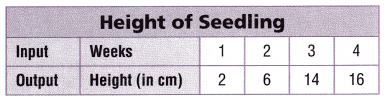Question 3.
Write ordered pairs to show the relationship between the week and the height.
Answer: The ordered pairs to show the relationship between the week and the height is (1, 2), (2, 6), (3, 14), (4, 16)

Question 4.
Graph the data in a coordinate grid.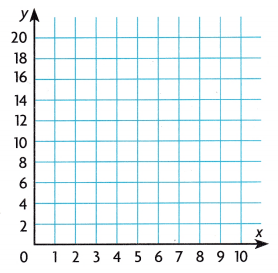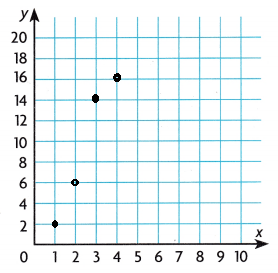Question 5.
Point A is 2 units to the right and 4 units up from the origin. What ordered pair describes point A? TEKS 5.8.A, 5.8.B
(A) (2, 0)
(B) (2, 4)
(C) (4, 2)
(D) (0, 4)

Question 6.
The input/output table shows Tori’s savings.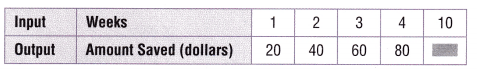If the x-coordinate is the number of weeks and the y-coordinate is the amount saved, which ordered pair represents the point showing the amount saved in 10 weeks? -jy TEKS 5.8.C
(A) (10, 100)
(B) (100, 10)
(C) (200, 10)
(D) (10, 200)

Question 7.
The rule for a pattern is y = 2x. Which point in the coordinate plane does NOT represent the pattern rule? TEKS 5.8.C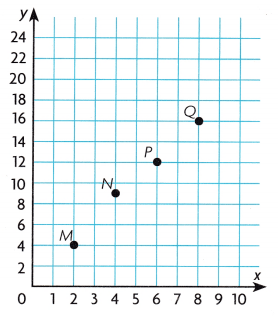(A) M
(B) N
(C) Q
(D) P